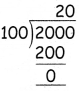Tamilnadu State Board New Syllabus Samacheer Kalvi 4th Maths Guide Pdf Term 3 Chapter 5 Money Ex 5.1 Textbook Questions and Answers, Notes.

## Tamilnadu Samacheer Kalvi 4th Maths Solutions Term 3 Chapter 5 Money Ex 5.1

Question 1.
In ₹ 1000 How many ₹ 500 notes are there? ________
How many ₹ 500 notes are there?  2

How many ₹ 200 notes are there? ________
How many ₹ 200 notes are there?   5How many ₹ 100 notes are there? ________
How many ₹ 100 notes are there?   10

How many ₹ 50 notes are there? ________
How many ₹ 50 notes are there?   20

Question 2.
Kamala had a change of ₹ 100, ₹ 50, ₹ 20 and ₹ 10 notes. How many rupees did she have in each to get the total of rupees 500?
₹ 500 = ₹ 100 ₹ 100 ₹ 100 ₹ 100 ₹ 50 ₹ 20 ₹ 20 ₹ 10
₹ 100 Note = 4
₹ 50 Note = 1
₹ 20 Note = 2
₹ 10 Note = 1Question 3.
Convert the following rupees into paise
(i) ₹ 7.50
(ii) ₹ 18.75
(iii) ₹ 54.68
(iv) ₹ 102.50
(v) ₹ 129.45
(vi) ₹ 308.61
(i) ₹ 7.50
₹ 7 = 7 × 100 = 700
50 paise
= 700 + 50
= 750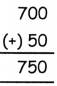(ii) ₹ 18.75
₹ 18 = 18 × 100 = 1800
75 paise
= 1800 + 75
= 1875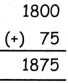(iii) ₹ 54.68
₹ 54 = 54 × 100 = 5400
68 paise
= 5400 + 68
= 5468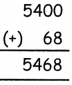(iv) ₹ 102.50
₹ 102 = 102 × 100 = 10200
50 paise
= 10200 + 50
= 10250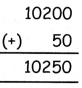(v) ₹ 129.45
₹ 129 = 129 × 100 = 12900
45 paise
= 12900 + 45
= 12945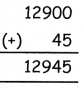(vi) ₹ 308.61
₹ 308 = 308 × 100 = 30800
61 paise
= 30800 + 61
= 30861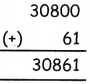Question 4.
(i) Write the denominator for the following amount.
₹ 466 = ₹ 200 ₹ 100 ₹ 100 ₹ 50 ₹ 10 ₹ 5 ₹ 1
₹ 200 × 1 = _____
₹ 100 × 2 = _____
₹ 50 × 1 = _____
₹ 10 × 1 = _____
₹ 5 × 1 = _____
₹ 1 × 1 = _____
Total = _____
₹ 200 × 1 =   ₹ 200
₹ 100 × 2 =  ₹ 200
₹ 50 × 1 =   ₹ 50
₹ 10 × 1 =   ₹ 10
₹ 5 × 1 =   ₹ 5
₹ 1 × 1 =   ₹ 1
Total =   ₹ 466(ii)
Use and fill in the boxes with the amounts given below, to get the given amount (₹ 845)
₹ 845 = ______ _______ _______ _______ _______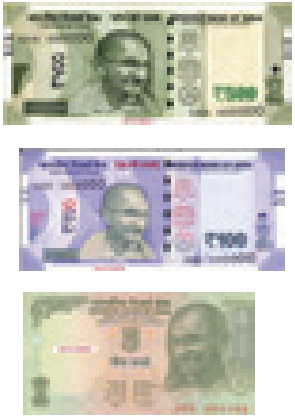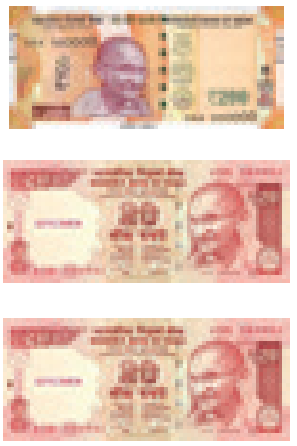₹ 845 = ₹ 500 ₹ 200 ₹ 100 ₹ 20 ₹ 20 ₹ 5

(iii)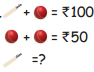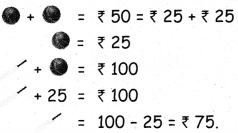(iv)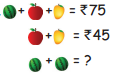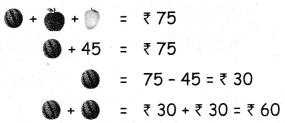Question 5.
Convert the following poise into rupees.
i. 800 paise
ii. 500 paise
iii. 2075 paise
iv. 6860 paise
v. 200 paise
vi. 150 paise
vii. 1000 paise
viii. 2000 paise
i. 800 paise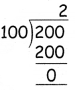ii. 500 paise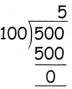iii. 2075 paise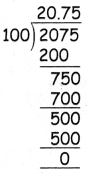iv. 6860 paise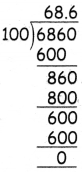v. 200 paise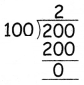vi. 150 paise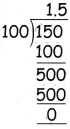vii. 1000 paise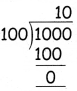viii. 2000 paise